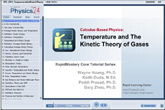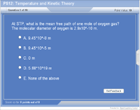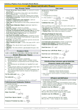How to Learn in 24 Hours?

 Need Help? M-F: 9am-5pm(PST): Toll-Free: (877) RAPID-10 or 1-877-727-4310 24/7 Online Technical Support: The Rapid Support Center vip@rapidlearningcenter.com Secure Online Order:Need Proof? Testimonials by Our Users

 Rapid Learning Courses: MCAT in 24 Hours (2021-22) USMLE in 24 Hours (Boards) Chemistry in 24 Hours Biology in 24 Hours Physics in 24 Hours Mathematics in 24 Hours Psychology in 24 Hours SAT in 24 Hours ACT in 24 Hours AP in 24 Hours CLEP in 24 Hours DAT in 24 Hours (Dental) OAT in 24 Hours (Optometry) PCAT in 24 Hours (Pharmacy) Nursing Entrance Exams Certification in 24 Hours eBook - Survival Kits Audiobooks (MP3)

 Tell-A-Friend: Have friends taking science and math courses too? Tell them about our rapid learning system.Home »  Physics »  Calculus Physics

Temperature and Kinetic Theory of Gases

 Topic Review on "Title": Kinetic Molecular Theory (KMT): An attempt to explain gas behavior.  Kelvin (K): Temperature scale used in gas calculations.  Has an absolute zero.   °C + 273 = K  Pressure: Force of gas molecules colliding with surfaces Atmospheric pressure: Pressure due to the layers of air in the atmosphere Standard Temperature and pressure: 1 atm (or anything it’s equal to) and 0°C (273 K) Ideal Gas: All assumptions of the kinetic molecular theory are true.  Molar Mass (Molecular Mass): Grams per mole for a molecule. Density: Mass per volume of a sample Average Translational Kinetic Energy (KEave): Average kinetic energy (energy due to motion) of each particle. Root-Mean-Square Speed (Vrms): One measure of average particle speed in a gas Maxwells Probability Distribution: Probability function that indicates the probability that a particle in a gas will be moving at a certain speed. Real Gas: Real gases have significant particle volume and significant attractions/repulsions Diffusion: The rate at which a gas travels through a container Effusion: The rate at which a gas escapes through a tiny hole

Rapid Study Kit for "Title":
 Flash Movie Flash Game Flash Card Core Concept Tutorial Problem Solving Drill Review Cheat Sheet"Title" Tutorial Summary : This tutorial describes gas in a variety of ways. Initially the role of temperature is described. As a gas is heated, it moves more and possesses more kinetic energy. It may expand depending upon the container. There are a variety of mathematical ways to describe the motion and behavior of gases. Avogadro’s, Boyles’, and Charles’ law may be transformed into the combined gas law. The ideal gas law further unifies these ideas into one useful formula package. Although not all gasses behave as if they were ideal, there are corrections that can be applied to create the real gas law. Finally, the concepts of diffusion and effusion are described. Respectively, these are the spreading of gas through space, or a tiny hole.

 Tutorial Features: Specific Tutorial Features: Graphic organizers to illustrate concept relationships. Example problems with notions and hints for difficult sections. Pneumonic memory device for the ideal gas law. Series Features: Concept map showing inter-connections of new concepts in this tutorial and those previously introduced. Definition slides introduce terms as they are needed. Visual representation of concepts Animated examplesworked out step by step A concise summary is given at the conclusion of the tutorial.

 "Title" Topic List: Temperature and kinetic energy of gasses Temperature Scales Fahrenheit Celsius Kelvin and absolute zero Kinetic Molecular Theory Assumptions Number of molecules Pressure Temperature Atmospheric pressure Pressure in a container Gas Laws and combined gas law Ideal gas law Molar mass and density Real gas law Molecular motion Diffusion and effusion

See all 24 lessons in high school  physics, including concept tutorials, problem drills and cheat sheets:
Teach Yourself Calculus Physics Visually in 24 Hours